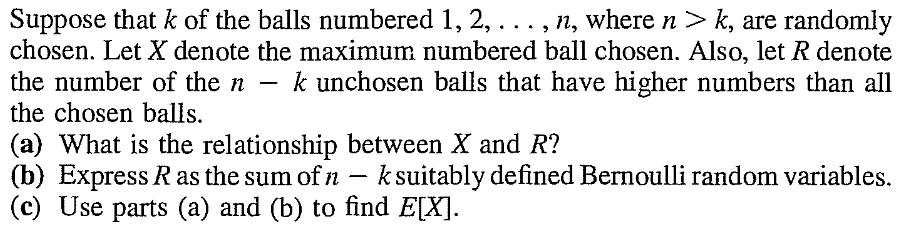[Home]

# Conditional distribution

Definition: Conditional distribution Let $X:\Omega\rightarrow S$ and $Y:\Omega\rightarrow T$ be joint distributed discrete random variables. Let $x\in S$ be some constant such that $P(X=x)> 0.$ Then the conditional distribution of $Y$ given $X=x$ is the probability distribution on $T$ $$A\mapsto P(Y\in A | X = x).$$

EXAMPLE: A 7-segment display shows any number from 0 to 9 at random (equal probabilities).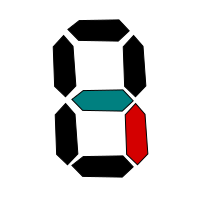Let $X$ be the indicator random variable of whether the blue segment is on. Similarly, $Y$ is the indicator for the red segment. Find the conditional distribution of $Y$ given $X.$

SOLUTION: Here $X,Y$ both take values in $\{0,1\}.$ We need to find $P(Y=y | X=x)$ for $x,y\in\{0,1\}.$

Now $P(Y=1|X=1) = P(X=1,Y=1)/P(X=1).$

Both the blue and the red segments are on in only the numbers 3,4,5,6,8,9. So $P(X=1,Y=1) = \frac{6}{10}.$

The blue segment is on in the numbers 2,3,4,5,6,8,9. So $P(X=1) =\frac{7}{10}.$

Hence $P(Y=1|X=1) = P(X=1,Y=1)/P(X=1) = \frac 67.$

You should now be able to work out the other three conditional probabilities similarly.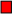We can define conditional CDF or conditional PMF in the obvious way.
Definition: Conditional expectation / variance Expectation (or variance) computed baed on a conditional distribution is called conditional expectation (variance).
It is important to understand that the conditional expectation/variance is a random variable, which is a function of the conditioning random variable.

## Unconditionals in terms of conditionals

Remember the throm of total probability: $$P(A) = P(B) P(A|B) + P(B^c)P(A|B^c),$$ where combined the two conditional probabilities of $A$ to arrive at the (unconditional) probability of $A?$

Well, we can do similar things with conditional expectation/variance also.
Tower property $E(Y) = E(E(Y|X)).$

Proof: Let $X$ take values $x_1,x_2,...$ and $Y$ take values $y_1,y_2,...$. Let the joint PMF of $(X,Y)$ be $$P(X=x_i~\&~Y=y_j) = p_{ij}.$$ Then $P(Y=y_j | X=x_i) = \frac{p_{ij}}{p_{i\bullet}}.$

So $E(Y|X=x_i) = \sum_j y_j \frac{p_{ij}}{p_{i\bullet}}.$

Expectation of this is $$\sum_i E(Y|X=x_i) p_{i\bullet} = \sum_i \sum_j y_j \frac{p_{ij}}{p_{i\bullet}}p_{i\bullet} = \sum_i \sum_j y_j p_{ij} = \sum_j y_j \sum_i p_{ij} = \sum_j y_j p_{\bullet j} = E(Y),$$ as required. [QED]

Many expectation problems can be handled step-bystep using this result. Here are some examples.

EXAMPLE: A casino has two gambling games:

1. Roll a fair die, and win Rs. $D$ if $D$ is the outcome.
2. Roll two fair dice, and win Rs 5 if both show the same number, but lose Rs 5 otherwise.
You throw a coin with $P(Head)=\frac 13$ and decide to play game 1 if $Head,$ and game 2 if $Tail.$ What is your expected gain?

SOLUTION: Let $X$ be your gain (in Rs), and let $Y$ be the outcome of the toss.

Then $E(X|Y=Head) = 3.5$ and $E(X|Y=Tail) = 5\times\frac{6-30}{36}=-\frac{10}{3}.$

So, by the tower property, $E(X) = P(X|Y=Head)\times P(Y=Head)+P(X|Y=Tail)\times P(Y=Tail) = \cdots.$The tower property is very useful for computing expectations involving a random number of random variables. Here is an example.

EXAMPLE: A random number $N$ of customers enter a shop in a day, where $N$ takes values in $\{1,...,100\}$ with equal probabilities. The $i$-th customer pays a random amount $X_i$, where $X_i$ takes values in $\{1,2,...,10+i\}$ ith equal probabilities. Assuming that $N,X_1,...,X_N$ are all independent, find the total expected payments by the customers on that day.

SOLUTION: We have $E(X_i) = \frac{11+i}{2}.$

So $E\left(\sum_1^N X_i|N\right) = \sum_1^N E(X_i|N) = \sum_1^N E(X_i) = \sum_1^N \frac{11+i}{2} = 5.5N+\frac{N(N+1)}{4}.$

By tower property, the required answer is $E\left(5.5N+\frac{N(N+1)}{4}\right)=\cdots.$EXAMPLE: 10 holes, numbered 1 to 10, in a row. 5 balls are dropped randomly in them (a hole may contain any number of balls). Call a ball "lonely" if there is no other ball in its hole or the adjacent holes. Find the expected number of lonely balls.

SOLUTION: Define the indicators $I_1,...,I_5$ as $$I_i = \left\{\begin{array}{ll}1&\text{if }i\mbox{-th ball is lonely}\\0&\text{otherwise.}\end{array}\right.$$ Then the total number of lonely balls is $X = \sum I_i.$

So we are to find $E(X) = \sum E(I_i).$

Let $Y_i =$ the hole where the $i$-th ball has fallen.

Then $E(I_i|Y_i=1)$ is the conditional probability that all the balls except the $i$-th one has landed in holes $2,...,10$ given that the $i$-th ball has landed in hole 1.

You should be able to compute this easily. Similarly, you can compute $E(I_i|Y_i=k)$ for $k=1,...,10.$

Notice that $Y_i$ can take values $1,...,10$ with equal probabilities.

So tower property should provide the answer as $$E(X) = \sum E(E(I_i|Y_i)) = \cdots.$$Theorem $V(Y) = E(V(Y|X)) + V(E(Y|X)).$

Proof: This follows directly from the tower property.

We know $$V(Y|X) = E(Y^2|X) - E^2(Y|X),$$ and hence $$E(V(Y|X)) = E(E(Y^2|X)) - E(E^2(Y|X)) = E(Y^2) - E(E^2(Y|X)).$$ Again, $$V(E(Y|X)) = E(E^2(Y|X)) - E^2(E(Y|X)) = E(E^2(Y|X)) - E^2(Y).$$ So $$E(V(Y|X)) + V(E(Y|X)) = E(Y^2)-E^2(Y) = V(Y),$$ as required. [QED]

## More than 2 variables

If $X,Y,Z$ are jointly distributed random variables, then we can talk about conditional distribution of $Z$ given $(X,Y)$ or $X$ given $Z$ or $(X,Z)$ given $Y,$ etc. We can even condition step by step. For example, we can talk about $E(E(Z|X,Y)|X).$ This is a function of $X$ alone.

## Substitution

Substition property Conditional distribution of $f(X,Y)$ given $X=x$ if same as the conditional distribution of $f(x,Y)$ given $X=x.$

Proof: This follows immediately from the definition of conditional probability. [QED]

# Problems for practice

1.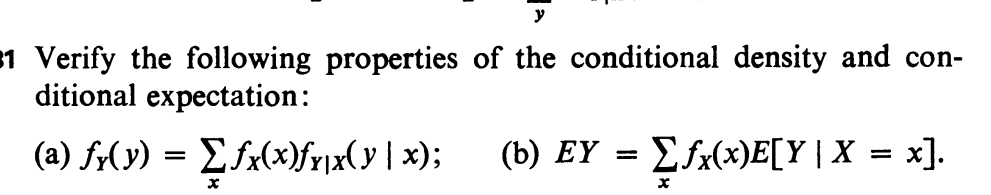2.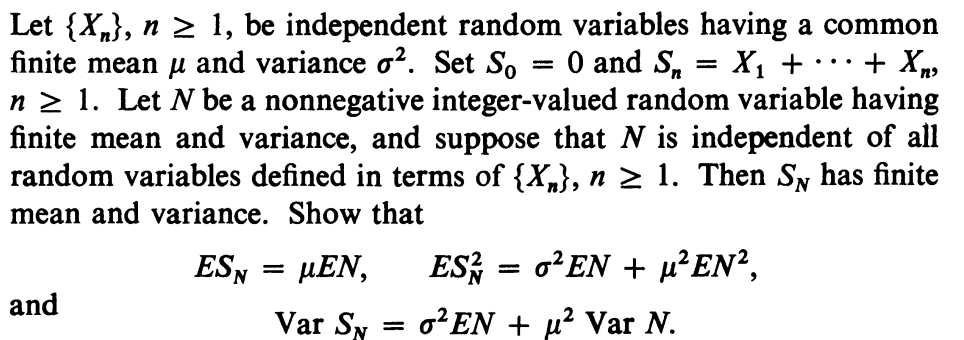3.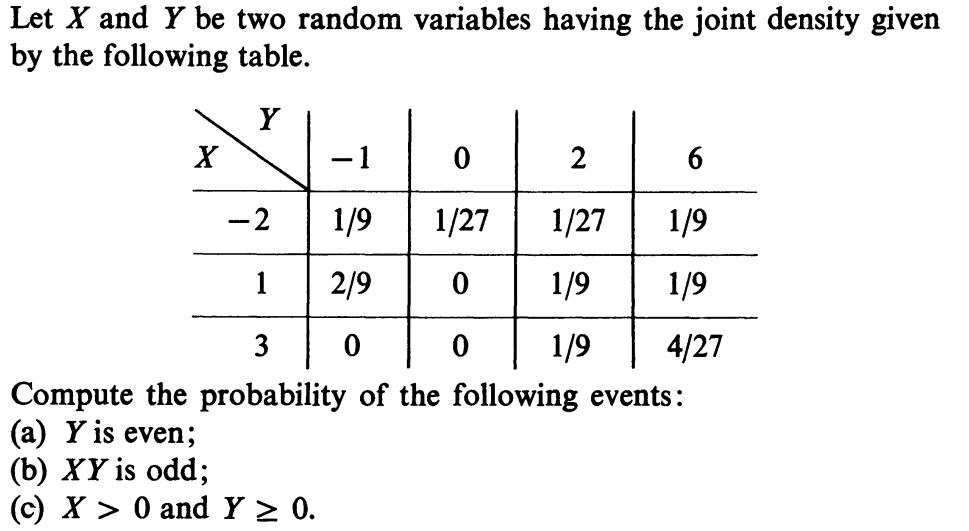4.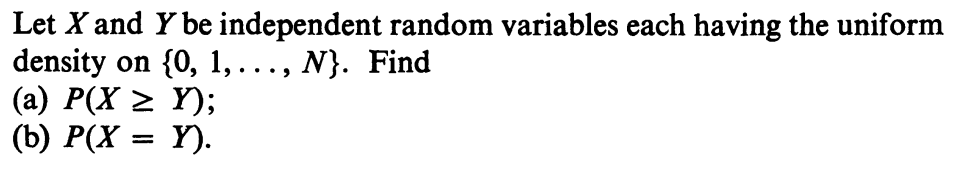5.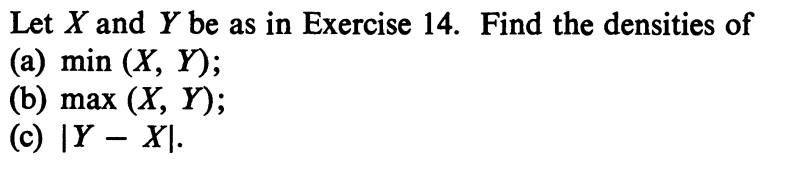6.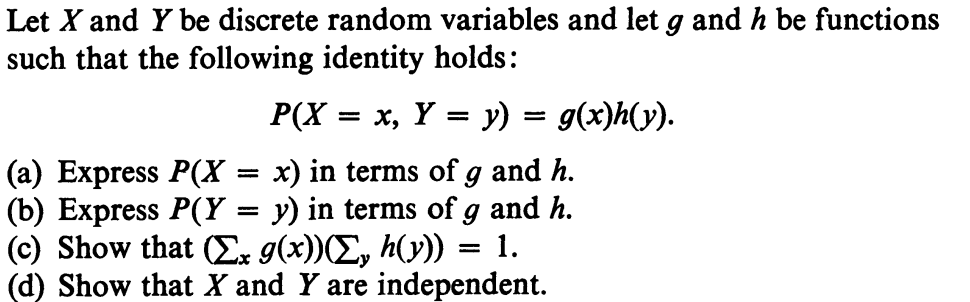7.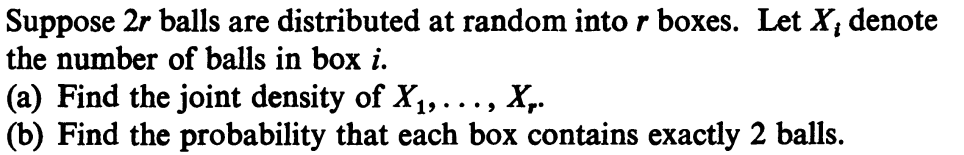8.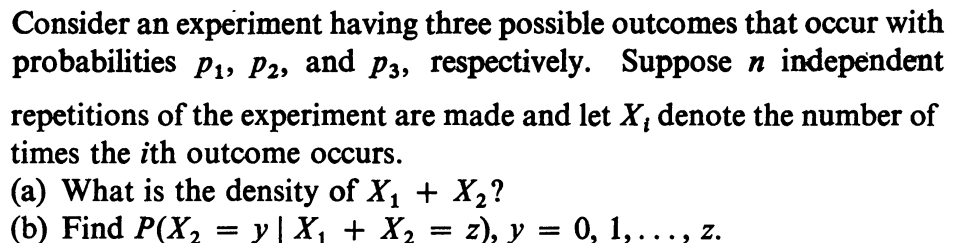9.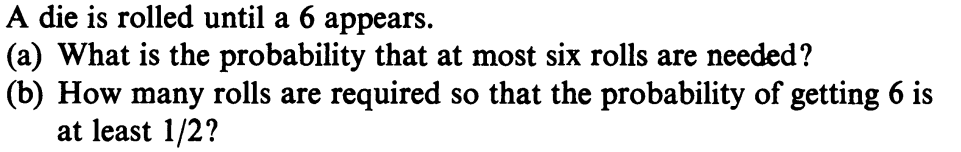10.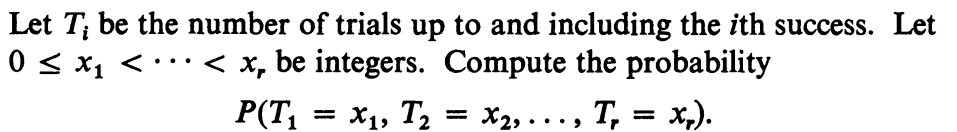11.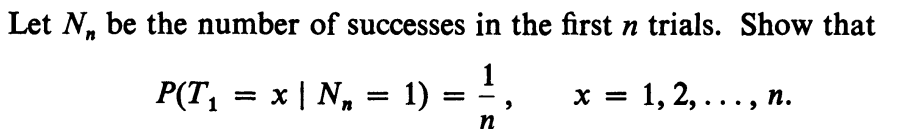12.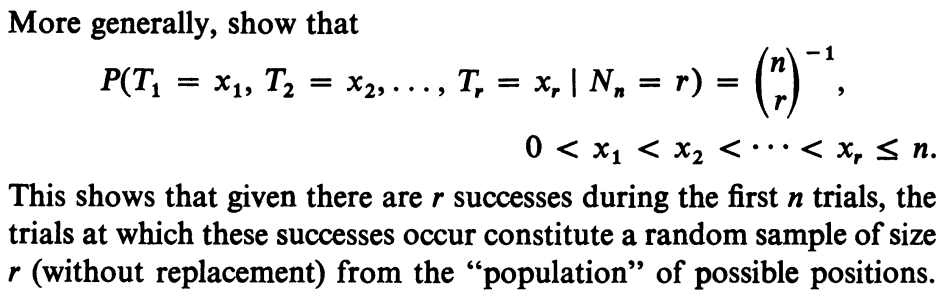13.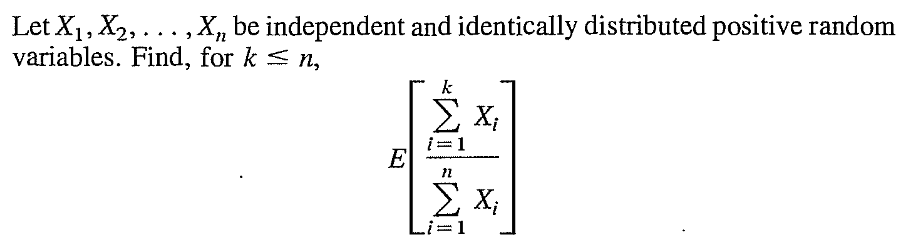14.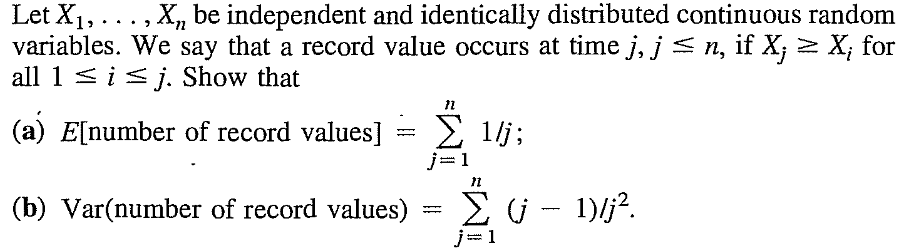Let $I_j$ be the indicator variable for whether there is a record at position $j.$ Then $P(I_j=1)$ may be computed by total probability: $$P(I_j=1) = \sum_{k=j}^n P(X_j=k)P(I_j=1|X_j=k).$$ Similarly for $P(I_jI_k=1).$
15.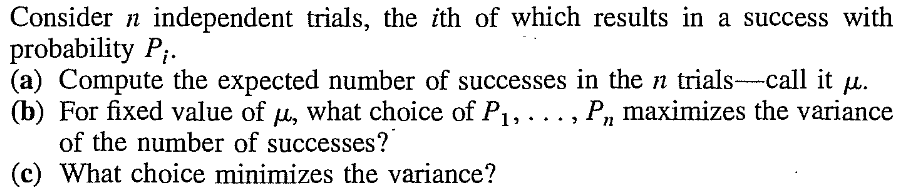The problem is basically optimising $\sum P_i^2$ subject to $\sum P_i$ being fixed. Cauchy-Scwartz might help.
16.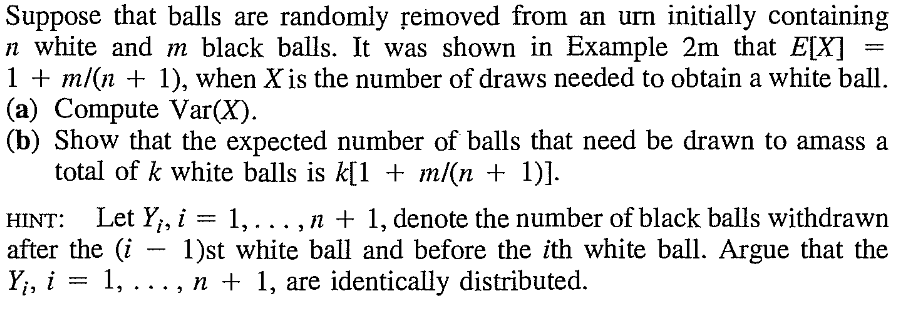This problem (from Ross) refers to Example 2m. Here is that example.
17.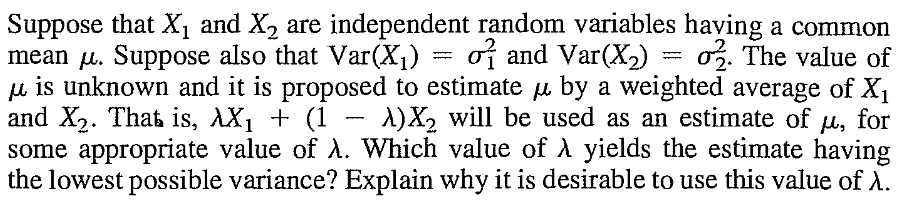18.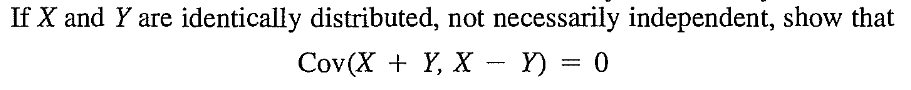19.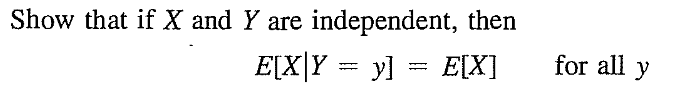20.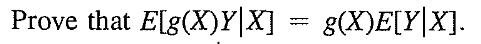21.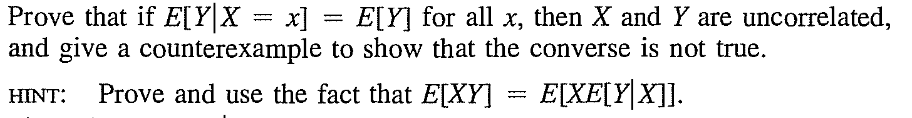22.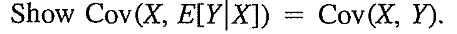23.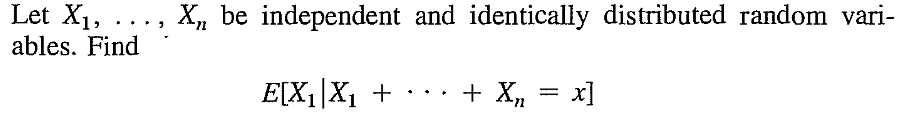24.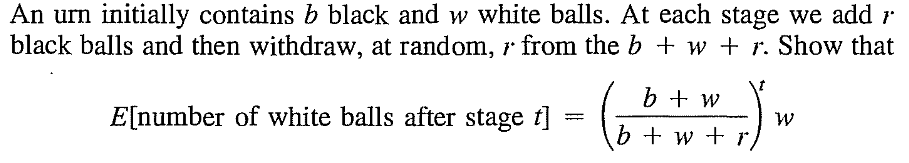25.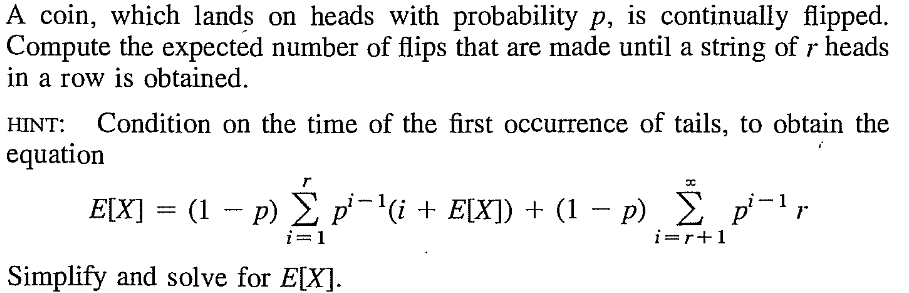26.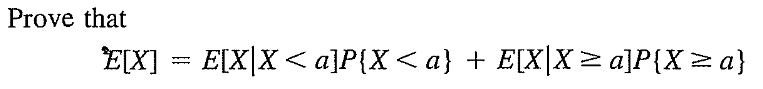27.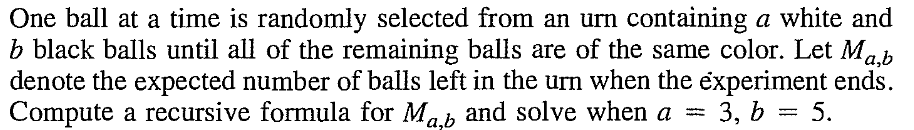28.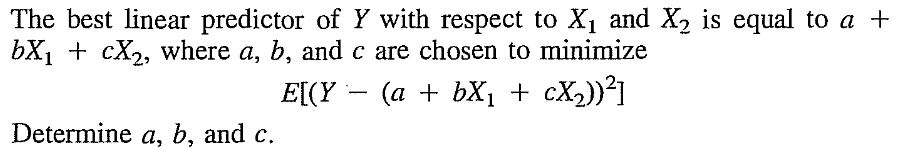29.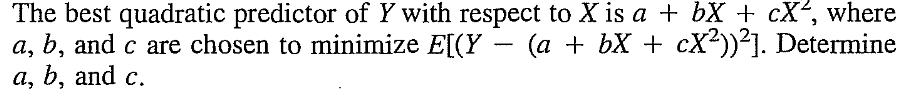30.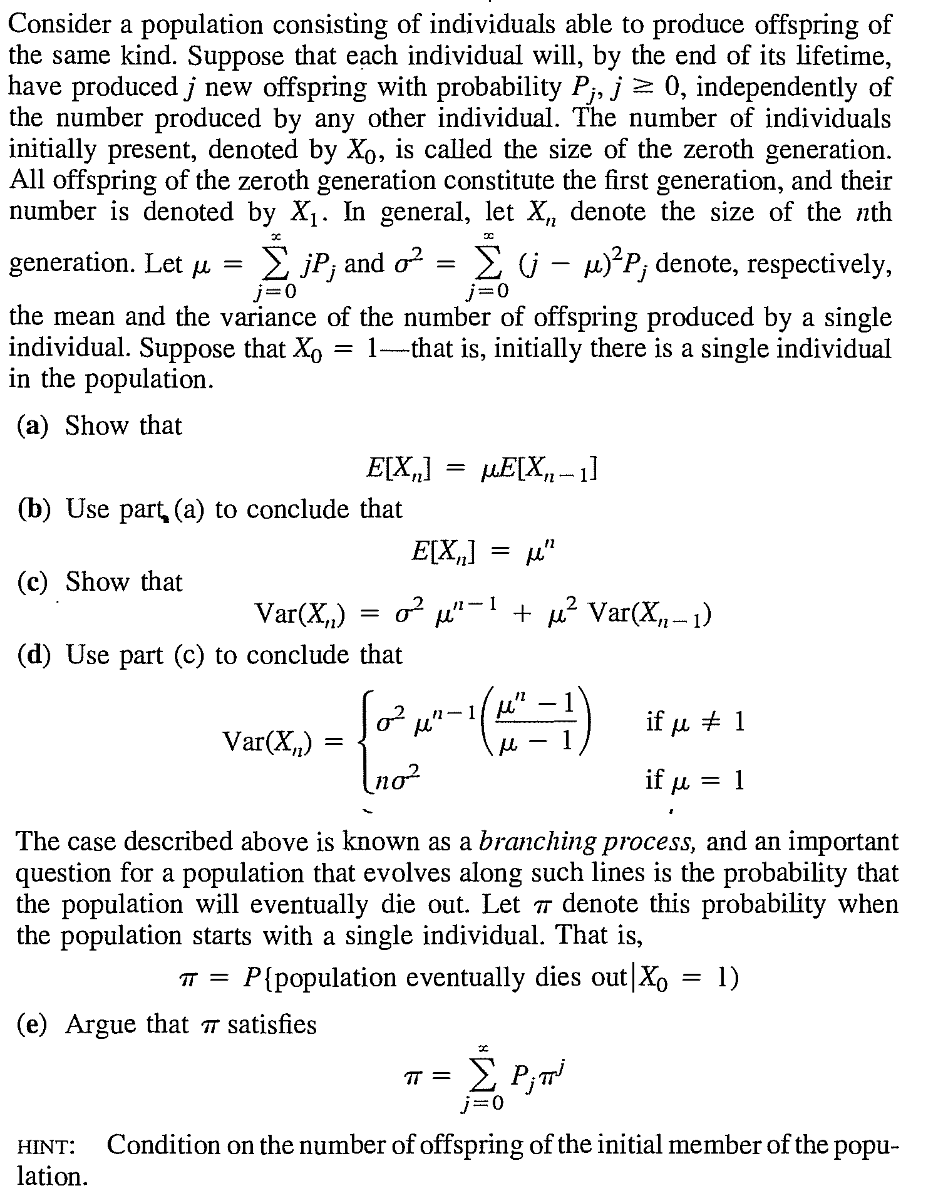31.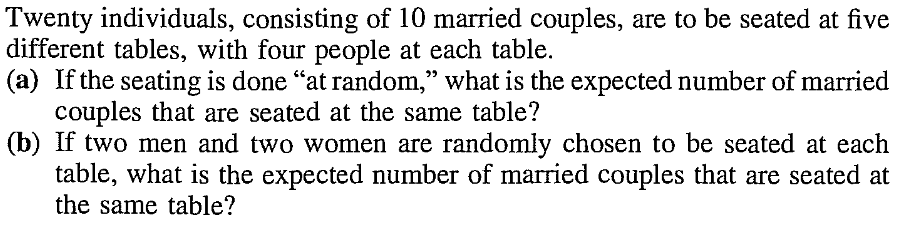32.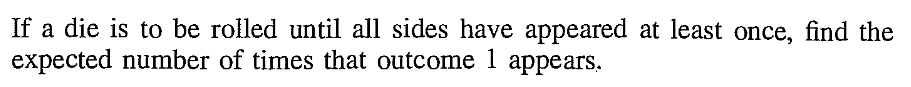33.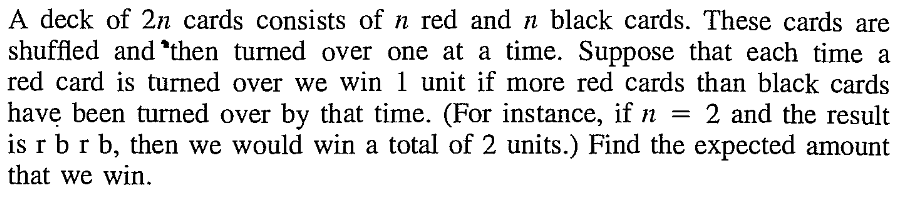34.